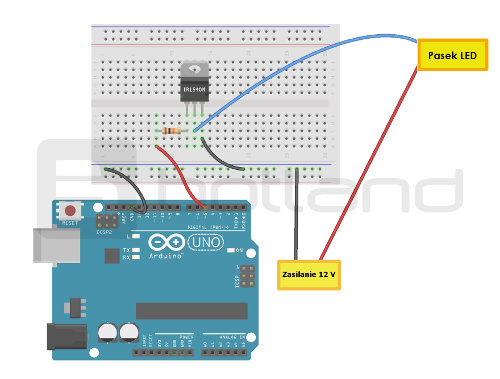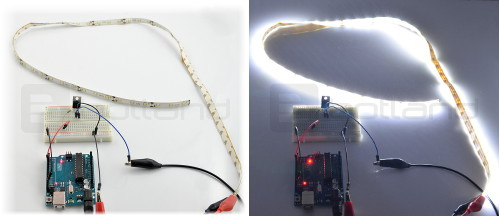# Control LED strip using Arduino

Using Arduino to control strips of LEDs. The above example shows connection method and shows how to load the sample program.

### Connection

The light is powered by a voltage of 12 V, while Arduino works with voltage of 5 V. to control them with the Arduino we will use the transistor as a switch. In this case IRL540N will be a good solution. Selected Arduino pin 5 is connected to the first leg of the transistor through a resistor of 10 ohms. The second is connected to "-" on the LEDS. The third to ground. Power +12 Volts connect your null-modem directly on the LED strip, while the mass of the entire system, we close with the mass of Arduino, as shown in the following figure.Connection diagram of the module.

#### Service

The presented system works in such a way that when we install pine 5 Arduino high status, the transistor begins to conduct the current supply and the INDICATOR. To this end, we use the program Blink from the Arduino examples (File -> Examples -> 01. Basics -> Blink).

Just have to change the Arduino pin 5, and we can set the delay time:

```// the setup function runs once when you press reset or power the board
void setup() {
// initialize digital pin 13 as an output.
pinMode(5, OUTPUT);
}

// the loop function runs over and over again forever
void loop() {
digitalWrite(5, HIGH); // turn the LED on (HIGH is the voltage level)
delay(3000); // wait for a second
digitalWrite(5, LOW); // turn the LED off by making the voltage LOW
delay(3000); // wait for a second
}

```

The program in turn ignites and extinguishes the LED strip every 3 seconds. The effect is visible in the photos below:With Fade (File -> Examples -> 01. Basics -> Fade) you can gradually lighten and darken the Led through PWM signal. We are mindful of making changes in the program Kontakt 5 (we also can przepiąć wire to pin 9. The main thing that this pin had a PWM denoted by a tilde). You can also experiment with other values.

```int led = 5; // the pin that the LED is attached to
int brightness = 0; // how bright the LED is
int fadeAmount = 5; // how many points to fade the LED by

// the setup routine runs once when you press reset:
void setup() {
// declare pin 5 to be an output:
pinMode(led, OUTPUT);
}

// the loop routine runs over and over again forever:
void loop() {
// set the brightness of pin 5:
analogWrite(led, brightness);

// change the brightness for next time through the loop: